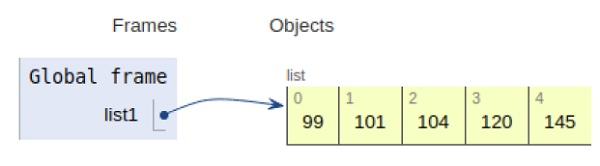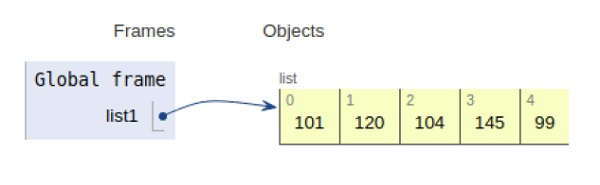# Python program to find the smallest number in a list

Problem statement − We are given al list, we need to display the smallest number available in the list

Here we can either sort the list and get the smallest element or use the built-in min() function to get the smallest element.

Now let’s observe the concept in the implementation below −

## Example

Live Demo

list1 = [101, 120, 104, 145, 99]
# sorting using built-in function
list1.sort()
print("Smallest element is:", list1)

## Output

Smallest element is: 99All the variables are declared in the local scope and their references are seen in the figure above.

## Example

Live Demo

list1 = [101, 120, 104, 145, 99]
#using built-in min fucntion
print("Smallest element is:", min(list1))

## Output

Smallest element is: 99All the variables are declared in the local scope and their references are seen in the figure above.

## Conclusion

In this article, we have learned about how we can find the smallest number in a list.